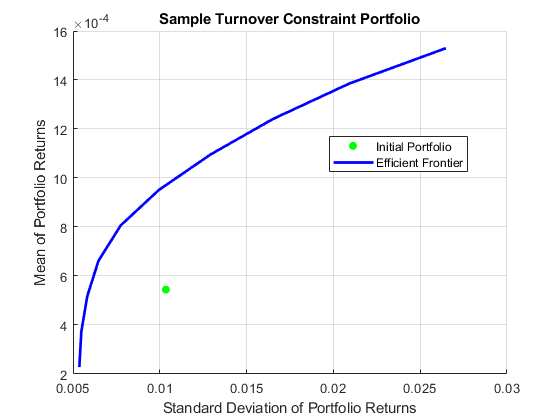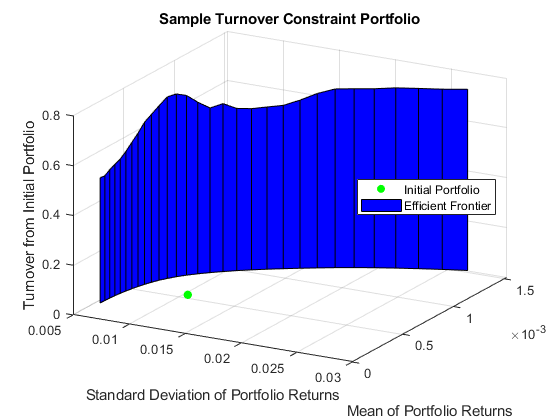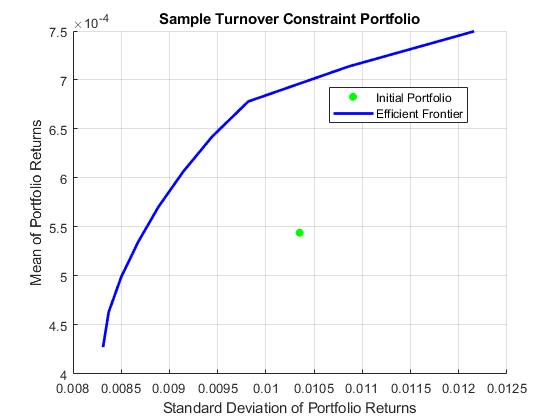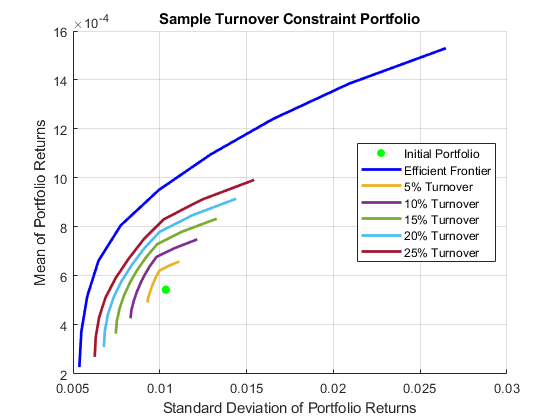Documentation

### This is machine translation

Mouseover text to see original. Click the button below to return to the English version of the page.

To view all translated materials including this page, select Country from the country navigator on the bottom of this page.

## Portfolio Analysis with Turnover Constraints

This example shows how to analyze the characteristics of a portfolio of equities, and then compares them with the efficient frontier. This example seeks to answer the question of how much closer can you get to the efficient frontier by only risking a certain percentage of a portfolio to avoid transaction costs.

### Import Data for the Portfolio Holdings

Load information on the current portfolio holdings from a Microsoft® Excel® spreadsheet into a table using the MATLAB® `readtable` function.

```AssetHoldingData = readtable('portfolio.xls'); % Create a normalized current holdings vector that shows the respective % investments as a percentage of total capital: W = AssetHoldingData.Value/sum(AssetHoldingData.Value); ```

### Import Market Data for Share Prices

Import the market data from a data source supported by Datafeed Toolbox™ that constitutes three years of closing prices for the stocks listed in the portfolio.

```addpath(fullfile(matlabroot,'examples','finance')); load SharePrices ```

### Create a `Portfolio` Object

The `Portfolio` class enables you to use the imported data to create a `Portfolio` object. The `estimateAssetMoments` function for the `Portfolio` object enables you to set up a portfolio given only a historical price or returns series. The `estimateAssetMoments` function estimates mean and covariance of asset returns from data even if there is missing data.

```P = Portfolio('Name', 'Sample Turnover Constraint Portfolio'); P = estimateAssetMoments(P,data,'DataFormat','Prices'); % You can assign text names to each asset in the portfolio. P = setAssetList(P,AssetHoldingData.Symbol); % Provide the current holdings. P = setInitPort(P,W); ```

### Perform Portfolio Optimization with No Turnover Constraint

The `Portfolio` object can optimize the holdings given any number of constraints. This example demonstrates using a simple, default constraint, that is, long positions only and 100% invested in assets.

```P = setDefaultConstraints(P); ```

Visualize this efficient frontier with the `plotFrontier` function.

```plotFrontier(P) ```### Visualize the Transaction Costs and Turnover

Due to transaction costs, it can be expensive to shift holdings from the current portfolio to a portfolio along this efficient frontier. The following custom plot shows that you must turn over between 50% and 75% of the holdings to get to this frontier.

```TurnoverPlot(P) ```### Perform Portfolio Optimization with a Turnover Constraint

How close can you get to this efficient frontier by only trading some of the portfolio? Assume that you want to trade only a certain percentage of the portfolio to avoid too much turnover in your holdings. This requirement imposes some nonlinear constraints on the problem and gives a problem with multiple local minima. Even so, the `Portfolio` object solves the problem, and you specify the turnover constraint using the `setTurnover` function.

```P10 = setTurnover(P,0.10); plotFrontier(P10) ```### Visualize the Efficient Frontier at Different Turnover Thresholds

This efficient frontier is much closer to the initial portfolio than the starting efficient frontier without turnover constraints. To visualize this difference, use the custom function `TurnoverConstraintPlot` to visualize multiple constrained efficient frontiers at different turnover thresholds.

```turnovers = 0.05:0.05:0.25; TurnoverConstraintPlot(P,turnovers) rmpath(fullfile(matlabroot,'examples','finance')); ```The `Portfolio` object is a powerful and efficient tool for performing various portfolio analysis tasks. In addition to turnover constraints, you can also optimize a `Portfolio` object for transaction costs for buying and selling portfolio assets using the `setCosts` function.